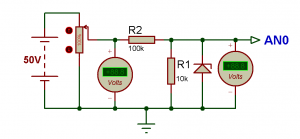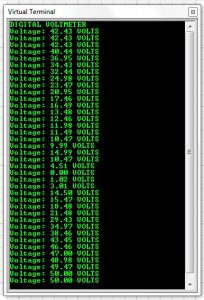# Digital voltmeter using Arduino UNO Range:0-50 volt Using SIMULINO UNO

This is a simple project showing you how to make a digital voltmeter using Arduino where the readings are displayed in a Liquid Crystal Display LCD20x4.

The proposed voltmeter design can read up to 50V. We are using analogue to digital conversion process.

Arduino microcontroller equipped with 10-bit analogue to digital converter (ADC). This means Arduino can read 2^10=1024 discrete voltage levels.In this project, we measure the input voltage range between 0 to 50V by using the voltage divider. It is very simple to use Arduino as a voltmeter. Arduino UNO has 5 analog pin to read the input analog value. The circuit consists of two resistors, one LCD display and an Arduino which is brain of the digital voltmeter. The two resistor acts as voltage divider, the node of the divider is connected to analogue pin # A0 of the Arduino, which reads the input voltage. Ground connection is established between Arduino and external voltage source.

You cannot feed 50V directly to a Arduino I/O pin, you need a resistor divider network that converts 0-50V range into 0-5V.A 5.1V Zener diode in the figure is to prevent V_AN0 (V in) to rise above 5.1V if the input voltage goes much above 50V. This will protect the Arduino board.The Arduino in built 10 bit ADC,can be used for measuring the 0Volt to 50 Volts Digital Voltmeter. LCD display connected with Arduino Uno will be used for displaying the measured voltage. This voltmeter can read only DC voltage.

## Code Designing in Arduino IDE 1.8.0 :

So, here’s the programming code youneed to use for Displaying voltage value on LCD using Arduino board in Proteus ISIS:

READ  Pressure Sensor BMP180 Interfacing with Arduino Uno

``` #include "LiquidCrystal.h" // initialize the library with the numbers of the interface pins // lcd(RS, E, d4, d5, d6, d7) LiquidCrystal lcd(2, 3, 4, 5, 6, 7); float voltage = 0.0; float V=0.0; float R1 = 100000.0; // resistance of R1 (100K) float R2 = 10000.0; // resistance of R2 (10K) int analog_value= 0; void setup() { Serial.begin(9600); Serial.println("DIGITAL VOLTMETER"); lcd.begin(20, 4); // set up the LCD's number of columns and rows lcd.setCursor (0,0); lcd.print(" LET'S THINK BINARY "); // Print a message to the LCD. lcd.setCursor (0,1); lcd.print(" www.electronify.org "); // Print a message to the LCD. lcd.setCursor(1,2); lcd.print("Digital Voltmeter"); } void loop() { // read the value at analog input pin A0 //and store it in the variable analog_value analog_value = analogRead(A0); voltage = (analog_value * 5.0) / 1024.0; V = voltage / (R2/(R1+R2)); if (V < 0.1) { V = 0.0;//statement to quash undesired reading ! } // Display the voltage in the serial monitor Serial.print ("Voltage: "); Serial.print (V); Serial.println (" VOLTS"); // Display the voltage in the LCD lcd.setCursor(0, 3); lcd.print("Voltage= "); lcd.print(V); lcd.setCursor(15,3); lcd.print("VOLTS"); // Wait 2 seconds between the measurements delay (2000); } ```

## Digital voltmeter using Arduino UNO Range:0-50 volt Using SIMULINO UNO (Schematic Diagram)

Results :

Compile the Arduino code and get the hex file from it.

For simulating with PROTEUS ISIS hit run button and then you will get following results:So, now we can see the LCD is displaying exactly the same values as are shown in the voltmeter. Now if you change the value of variable resistor then the value of voltage will change in LCD.

READ  Mint-Sized Success Meter (quit smoking!) with Arduino

This digital voltmeter using Arduino UNO board can read voltage only between 0-50 volt.

You candownload the Source Code and Proteus files etc from here arduino digital voltmeter:
This Our Group (thanks to join and participate) : https://www.facebook.com/groups/816450708460518/

Youtube Channel (thanks to subscribe) :

#### This Post / Project can also be found using search terms:

• how to make a digital voltmeter
• simulino mega
• arduino simulino uno
• Arduino Uno Schematic Diagram